# Basics of GIS¶

Authors:

Shammunul Islam (shais13irs@gmail.com)

## 1. Basic data types in GIS¶

There are 2 basic types of geospatial data:

1. Vector data

2. Raster data

For vector data, there are 3 basic data types:

a. Point

b. LineString

c. Polygon

### Point data¶

In [ ]:
from shapely.geometry import Point

In [ ]:
p1 = Point(13, 27.21)
p1

Out[ ]:

The code is importing the Point class from the shapely.geometry module. The Point class is a geometric object that represents a point in 2D space with x and y coordinates.

Then, the code creates a new Point object named p1 by calling the Point constructor with the arguments (13, 27.21). This creates a point at the coordinates (13, 27.21) in 2D space.

Similarly, we can create a second or multiple points.

In [ ]:
p2 = Point(61, 56.21)
p2

Out[ ]:
In [ ]:
p3 = Point(30, -6.21)
p3

Out[ ]:
In [ ]:
p4 = Point(2, -30.21)
p4

Out[ ]:

### LineString¶

In [ ]:
from shapely.geometry import LineString

In [ ]:
line1 = LineString([p1, p2])
line1

Out[ ]:

The code creates a LineString object named line1 using the LineString class from the shapely.geometry module.

The LineString object represents a line in 2D space as a sequence of points. The LineString constructor takes a list of Point objects as its argument, so the code is passing a list containing two Point objects p1 and p2 as the argument to create a line segment connecting these two points.

In [ ]:
line2 = LineString([p1, p2, p3])
line2

Out[ ]:

### Polygon¶

In [ ]:
from shapely.geometry import Polygon

In [ ]:
Polygon([p1, p2, p3])

Out[ ]:
In [ ]:
Polygon([p1, p2, p3, p4])

Out[ ]:

We can have as many points as we need or want for polygons.

### Multipoints¶

The MultiLineString class in Python's Shapely library is used to represent collections of line objects in two-dimensional space. A MultiLineString object is a collection of one or more LineString objects that are considered as a single entity.

Example: dataset of the locations of weather stations in Bangladesh.

In geospatial data analysis, the MultiPoint class in Python's Shapely library is used to represent collections of point objects in two-dimensional space. A MultiPoint object is a collection of one or more Point objects that are considered as a single entity.

In [ ]:
from shapely.geometry import MultiPoint, MultiLineString, MultiPolygon

In [ ]:
multip1 = MultiPoint([p1, p2, p3, p4])
multip1

Out[ ]:

### MultiLineString¶

The MultiLineString class in Python's Shapely library is used to represent collections of line objects in two-dimensional space. A MultiLineString object is a collection of one or more LineString objects that are considered as a single entity.

Example: dataset of roads in Dhaka.

### MultiPolygon¶

The MultiPolygon class in Python's Shapely library is used to represent collections of polygon objects in two-dimensional space. A MultiPolygon object is a collection of one or more Polygon objects that are considered as a single entity.

Example: dataset of buildings footprints in New York City.

Let's create two polygons poly1 and poly2 from the four points we defined above and consider these polygons as a single entity -- a MultiPolygon.

In [ ]:
#  imports the MultiPolygon class from the shapely.geometry module
from shapely.geometry import MultiPolygon

In [ ]:
poly1 = Polygon([p1, p2, p3])
poly2= Polygon([p2, p3, p4])
multi_poly = MultiPolygon([poly1, poly2])
multi_poly

Out[ ]:
In [ ]:
poly1

Out[ ]:
In [ ]:
poly2

Out[ ]:
In [ ]:
type(multi_poly)

Out[ ]:
shapely.geometry.multipolygon.MultiPolygon

With all the above objects, we can use the function type to see or check their data type.

## 2. Getting coordinates¶

### Coordinate of a point¶

In [ ]:
p1.coords

Out[ ]:
<shapely.coords.CoordinateSequence at 0x7f2fc8dba230>
In [ ]:
coordinates = list(p1.coords)
coordinates

Out[ ]:
[(13.0, 27.21)]

We can see that the coordinates are given as a list of tuple. The first value is the x-cordinate and the second value is the y-cordiante. Latitude is the x-cordinate and longitude is the y-coordinate.

### Getting latitude and longitude¶

• First way using coords
In [ ]:
# latitude
coordinates = list(p1.coords)
coordinates

Out[ ]:
13.0
In [ ]:
# longitude
coordinates

Out[ ]:
27.21
• Second way using x and y attribute of the Point object
In [ ]:
# latitude
p1.x

Out[ ]:
13.0
In [ ]:
# longitude
p1.y

Out[ ]:
27.21

### Coordinate of a LineString¶

• Using coords
In [ ]:
line2.coords

Out[ ]:
<shapely.coords.CoordinateSequence at 0x7f2fc8d39210>
In [ ]:
coordinates = list(line2.coords)
coordinates

Out[ ]:
[(13.0, 27.21), (61.0, 56.21), (30.0, -6.21)]

It shows the coordinates of the points (here, three points) used to create this line.

To get the latitude of the third point, we can access it using the coordinates list in the following way:

In [ ]:
coordinates

Out[ ]:
30.0
• Using xy

The xy attribute of a LineString object in Python's Shapely library returns a tuple containing two arrays representing the x and y coordinates of the line vertices.

In [ ]:
line2.xy

Out[ ]:
(array('d', [13.0, 61.0, 30.0]), array('d', [27.21, 56.21, -6.21]))
In [ ]:
# Getting longitude of the third point
line2.xy

Out[ ]:
-6.21

### Coordinate of a Polygon¶

In [ ]:
poly1.exterior

Out[ ]:
In [ ]:
list(poly1.exterior.coords)

Out[ ]:
[(13.0, 27.21), (61.0, 56.21), (30.0, -6.21), (13.0, 27.21)]

The bounds attribute of a Polygon or MultiPolygon object in Python's Shapely library returns a tuple containing the minimum and maximum values of the x and y coordinates that define the bounding box of the polygon(s).

## 3. Getting length¶

### Length of line¶

In [ ]:
line1.length

Out[ ]:
56.08029957123981

### Length of polygon¶

In [ ]:
poly1.length

Out[ ]:
163.2696044376992

## 4. Getting area¶

### Area of a polygon¶

In [ ]:
poly1.area

Out[ ]:
1048.58

## 5. Centroid¶

The centroid of LineString or Polygon is a point representing the "center of mass" or "average position" of the object. The centroid is a useful measure of the location and shape of the object.

In Shapely, the centroid property of a LineString or Polygon object returns a Point object that represents the centroid of the object. This Point object has an x and y coordinate.

### Centroid of a LineString¶

In [ ]:
line1.centroid

Out[ ]:
In [ ]:
print(line1.centroid)

POINT (37.00000000000001 41.71)


### Centroid of a Polygon¶

In [ ]:
poly1.centroid

Out[ ]:
In [ ]:
print(poly1.centroid)

POINT (34.666666666666664 25.736666666666668)


## 6. Distance¶

Distance from point 1 (p1) to point 2 (p2).

In [ ]:
p1.distance(p2)

Out[ ]:
56.08029957123981

Distance from point 3 (p3) to point 1 (p1).

In [ ]:
p3.distance(p1)

Out[ ]:
37.49528503692164

## 7. Buffer¶

• Buffer around a Point object or geometry
In [ ]:
p1.buffer(33)

Out[ ]:
• Buffer around a MultiPoint object or geometry
In [ ]:
multip1.buffer(14)

Out[ ]:
• Buffer around a LineString object or geometry
In [ ]:
line1.buffer(12)

Out[ ]:
• Buffer around a Polygon object or geometry
In [ ]:
poly1.buffer(8)

Out[ ]:
In [ ]:
# just to see poly1 without buffer
poly1

Out[ ]:

## 8. Contains¶

The contains method is a Boolean function from the Shapely library that returns True if the point is inside the polygon, and False otherwise.

In [ ]:
poly1.contains(p3) # p3 is one of the corners of this polygon

Out[ ]:
False
In [ ]:
new_point = Point(32, 28)

In [ ]:
# plot the Polygon and Point objects together
import matplotlib.pyplot as plt
fig, ax = plt.subplots()
ax.set_aspect('equal')
ax.plot(*poly1.exterior.xy)
ax.plot(new_point.x, new_point.y, 'o', color='red')
plt.show()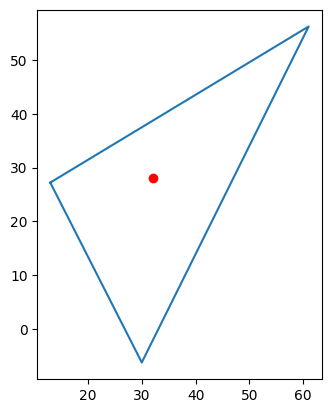In [ ]:
poly1.contains(new_point)

Out[ ]:
True

Create a new point that is outside the polygon boundary.

In [ ]:
new_point2 = Point(25, 45)

In [ ]:
# plot the Polygon and Point objects together
fig, ax = plt.subplots()
ax.set_aspect('equal')
ax.plot(*poly1.exterior.xy)
ax.plot(new_point2.x, new_point2.y, 'o', color='red')
plt.show()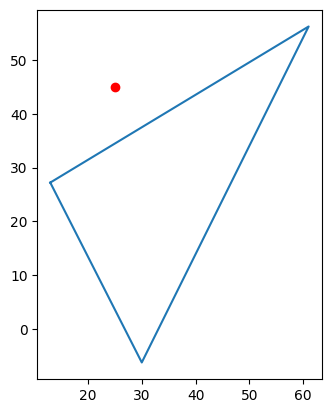In [ ]:
poly1.contains(new_point2)

Out[ ]:
False

As expected, it says that this is out of the boundary of this polygon.

## 9. Within¶

This is similar to contains with the difference that it checks whether a object is within the boundary of another object.

In [ ]:
new_point.within(poly1)

Out[ ]:
True
In [ ]:
new_point2.within(poly1)

Out[ ]:
False

## 10. Crosses¶

As the name suggests, crosses allows us to see whether a geometry object (here, LineString) crosses another geometry object (here, a Polygon).

In [ ]:
from shapely.geometry import Point, Polygon, LineString
import matplotlib.pyplot as plt

# create multiple Point objects to be utilized for creating a Polygon object
p1 = Point(13, 27.21)
p2 = Point(61, 56.21)
p3 = Point(30, -6.21)
# use these points to create Polygon object
poly1 = Polygon([p1, p2, p3])

# create a Point object
point = Point(50, 6)

# create two LineString objects
line1 = LineString([(32, 28), (25, 45)])
line2 = LineString([(40, -5), (50, 20)])

# plot the Polygon, Point, and LineString objects together
fig, ax = plt.subplots()
ax.set_aspect('equal')
ax.plot(*poly1.exterior.xy)
ax.plot(point.x, point.y, 'o', color='red')
ax.plot(*line1.xy, color='blue')
ax.plot(*line2.xy, color='orange')
plt.show()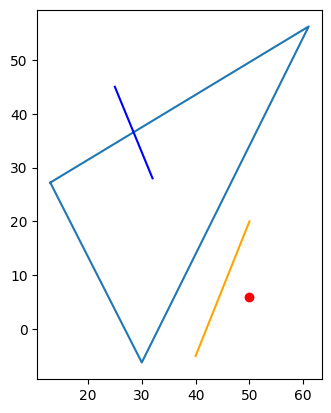We see that line1 or blue line crosses the polygon. How can we check this without checking visually? The answer is crosses.

In [ ]:
line1.crosses(poly1)

Out[ ]:
True

The result is as expected. Now, does the line line2 with orange color crosses the polygon? We know it doesn't, let's check this by using crosses again.

In [ ]:
line2.crosses(poly1)

Out[ ]:
False

## 11. Difference¶

The difference() method is used to compute the difference between two geometric objects. When this method is called on a Polygon object, it returns a new Polygon object that represents the difference between the original Polygon object and another Polygon object passed as an argument to the difference() method.

In [ ]:
p1 = Point([20, 40])

In [ ]:
p2 = Point([40, 80])

In [ ]:
from shapely.geometry import Point
import matplotlib.pyplot as plt

# create two Point objects
point1 = Point(0.5, 0.5)
point2 = Point(1, 1)

# plot the Point objects together
fig, ax = plt.subplots()
ax.set_aspect('equal')
ax.plot(point1.x, point1.y, 'o', color='red')
ax.plot(point2.x, point2.y, 'o', color='blue')
plt.show()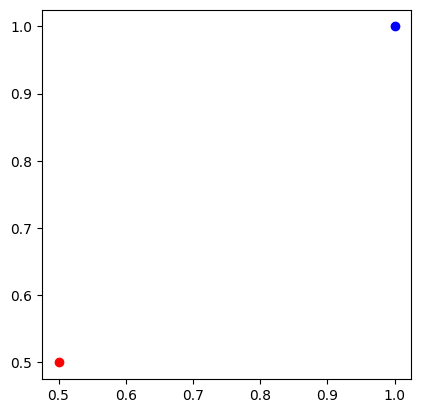In [ ]:
from shapely.geometry import Point, Polygon
import matplotlib.pyplot as plt

# create two Point objects
point1 = Point(0.5, 0.5)
point2 = Point(1, 1)

# create Polygon objects with buffer zone around each point
buffer1 = point1.buffer(0.6)
buffer2 = point2.buffer(0.6)

# plot the Polygon objects with buffer zone
fig, ax = plt.subplots()
ax.set_aspect('equal')
ax.fill(*buffer1.exterior.xy, alpha=0.5, color='red')
ax.fill(*buffer2.exterior.xy, alpha=0.5, color='blue')
plt.show()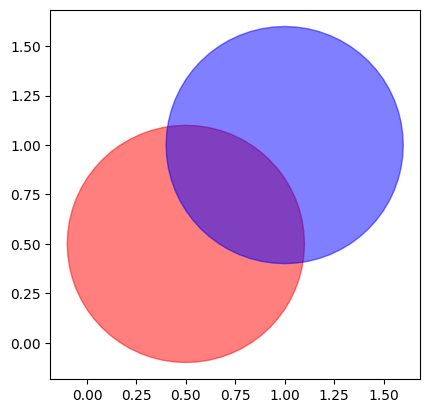In [ ]:
result = buffer1.difference(buffer2)

import matplotlib.pyplot as plt
from shapely.geometry import Point

# plot the difference between buffer1 and buffer2
fig, ax = plt.subplots()
ax.set_aspect('equal')
# ax.axis('off')
ax.fill(*result.exterior.xy, alpha=0.5, color='red')
plt.show()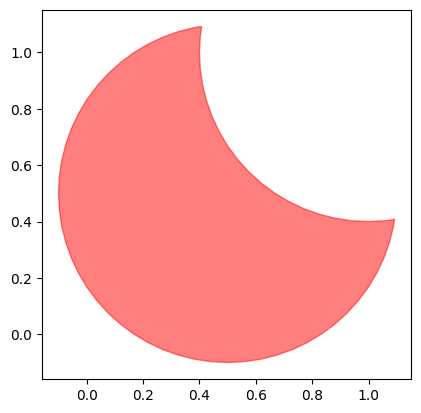In [ ]:
result2 = buffer2.difference(buffer1)

import matplotlib.pyplot as plt
from shapely.geometry import Point

# plot the difference between buffer2 and buffer1
fig, ax = plt.subplots()
ax.set_aspect('equal')
# ax.axis('off')
ax.fill(*result2.exterior.xy, alpha=0.5, color='blue')
plt.show()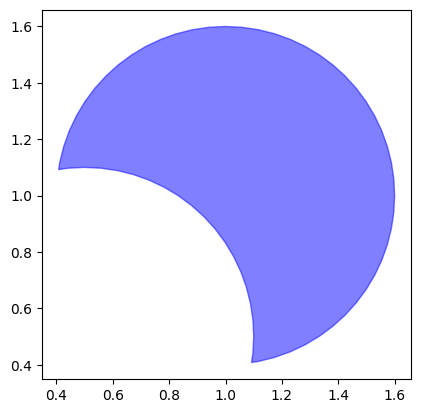In [ ]:



## 12. Intersection¶

intersection is used to obtain the region where two or more geometries overlap. When we apply the intersection method to two geometries, the resulting geometry is a new geometry that represents the region where the two original geometries overlap. The intersection method can be applied to any type of geometry, including Points, Lines, and Polygons.

In [ ]:
intersection = buffer1.intersection(buffer2)

# plot the intersection between buffer1 and buffer2
fig, ax = plt.subplots()
ax.set_aspect('equal')
# ax.axis('off')
ax.fill(*intersection.exterior.xy, alpha=0.5, color='red')
plt.show()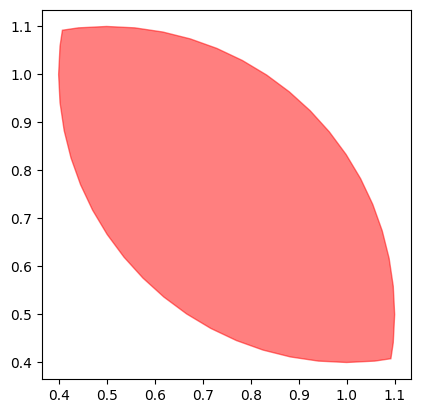## 13. Union¶

We use union to combine two or more geometries into a single geometry. When we apply the union method to two geometries, it results into new geometry that encompasses the area covered by both of the original geometries. We can use the union method with Points, Lines, and Polygons.

In [ ]:
union = buffer1.union(buffer2)

# plot the union of buffer1 and buffer2
fig, ax = plt.subplots()
ax.set_aspect('equal')
# ax.axis('off')
ax.fill(*union.exterior.xy, alpha=0.5, color='red')
plt.show()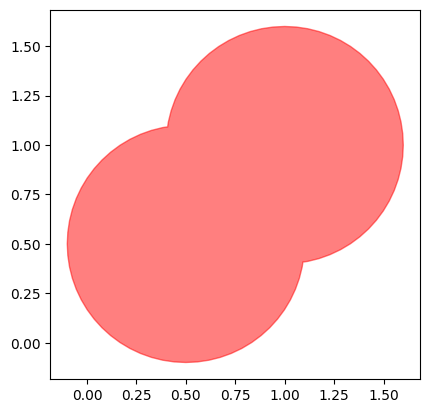## 14. Symmetric difference¶

The symmetric_difference() method in the Shapely library computes the symmetric difference between two geometric objects. It returns a new geometric object that represents the area of the geometries that do not overlap.

In [ ]:
union = buffer1.symmetric_difference(buffer2)

# plot the union of buffer1 and buffer2
fig, ax = plt.subplots()
ax.set_aspect('equal')
# ax.axis('off')
for polygon in union.geoms:
ax.fill(*polygon.boundary.xy, alpha=0.5, color='red')
plt.show()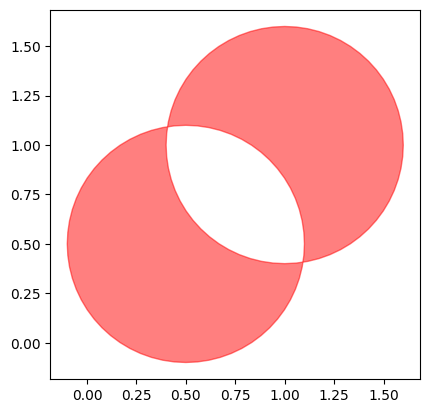## 15. Plot a shapefile¶

We will be using GeoPandas which is basically a Pandas dataframe plus GeoSeries which is a column that contains vector geometry. These geometries are handled as Shapely object and thus the operations that we have already done for different Shapely object can also be applied to GeoPandas geometries.

In [ ]:
from google.colab import drive
drive.mount('/content/drive')

Drive already mounted at /content/drive; to attempt to forcibly remount, call drive.mount("/content/drive", force_remount=True).

In [ ]:
# Put the path to the shapefile in your Google Drive here
path = "/content/drive/MyDrive/Geospatial_Python/Data/"

In [ ]:
!pip install geopandas

Looking in indexes: https://pypi.org/simple, https://us-python.pkg.dev/colab-wheels/public/simple/
Requirement already satisfied: geopandas in /usr/local/lib/python3.10/dist-packages (0.12.2)
Requirement already satisfied: fiona>=1.8 in /usr/local/lib/python3.10/dist-packages (from geopandas) (1.9.3)
Requirement already satisfied: packaging in /usr/local/lib/python3.10/dist-packages (from geopandas) (23.1)
Requirement already satisfied: pandas>=1.0.0 in /usr/local/lib/python3.10/dist-packages (from geopandas) (1.5.3)
Requirement already satisfied: pyproj>=2.6.1.post1 in /usr/local/lib/python3.10/dist-packages (from geopandas) (3.5.0)
Requirement already satisfied: shapely>=1.7 in /usr/local/lib/python3.10/dist-packages (from geopandas) (1.8.5.post1)
Requirement already satisfied: certifi in /usr/local/lib/python3.10/dist-packages (from fiona>=1.8->geopandas) (2022.12.7)
Requirement already satisfied: click-plugins>=1.0 in /usr/local/lib/python3.10/dist-packages (from fiona>=1.8->geopandas) (1.1.1)
Requirement already satisfied: attrs>=19.2.0 in /usr/local/lib/python3.10/dist-packages (from fiona>=1.8->geopandas) (23.1.0)
Requirement already satisfied: cligj>=0.5 in /usr/local/lib/python3.10/dist-packages (from fiona>=1.8->geopandas) (0.7.2)
Requirement already satisfied: munch>=2.3.2 in /usr/local/lib/python3.10/dist-packages (from fiona>=1.8->geopandas) (2.5.0)
Requirement already satisfied: click~=8.0 in /usr/local/lib/python3.10/dist-packages (from fiona>=1.8->geopandas) (8.1.3)
Requirement already satisfied: numpy>=1.21.0 in /usr/local/lib/python3.10/dist-packages (from pandas>=1.0.0->geopandas) (1.22.4)
Requirement already satisfied: pytz>=2020.1 in /usr/local/lib/python3.10/dist-packages (from pandas>=1.0.0->geopandas) (2022.7.1)
Requirement already satisfied: python-dateutil>=2.8.1 in /usr/local/lib/python3.10/dist-packages (from pandas>=1.0.0->geopandas) (2.8.2)
Requirement already satisfied: six in /usr/local/lib/python3.10/dist-packages (from munch>=2.3.2->fiona>=1.8->geopandas) (1.16.0)

In [ ]:
import geopandas as gpd
import matplotlib.pyplot as plt

# Plot the shapefile
bd.plot()

# Show the plot
plt.show()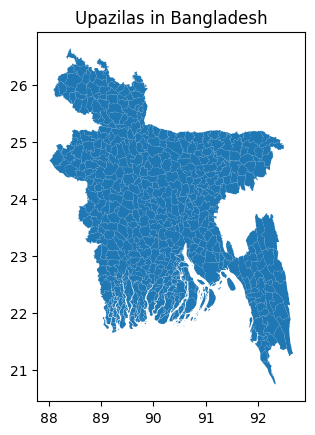We can also zoom in to the northeast part of Bangladesh by using cx property of the GeoPandas dataframe. Here, we are specifying the range for latitude and longitude.

In [ ]:
bd.cx[91:92.5, 24:25.2].plot()

Out[ ]:
<Axes: >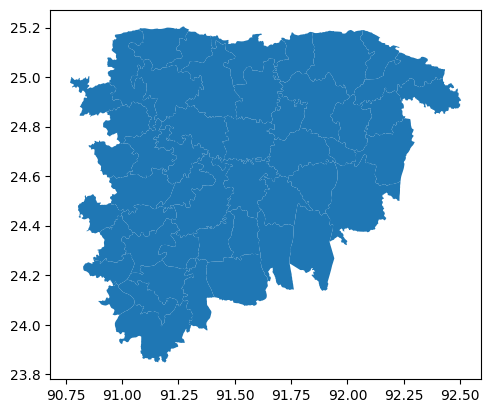Let's have a look at the content of this GeoPandas dataframe.

In [ ]:
bd.head()

Out[ ]:
ID_0 ISO NAME_0 ID_1 NAME_1 ID_2 NAME_2 ID_3 NAME_3 TYPE_3 ENGTYPE_3 NL_NAME_3 VARNAME_3 geometry
0 20 BGD Bangladesh 1 Barisal 1 Barisal 1 Agailjhara Upazila|Thana|Po Sub-district NaN NaN POLYGON ((90.13228 23.02925, 90.13877 23.03056...
1 20 BGD Bangladesh 1 Barisal 1 Barisal 2 Babuganj Upazila|Thana|Po Sub-district NaN NaN POLYGON ((90.27067 22.73984, 90.26520 22.74118...
2 20 BGD Bangladesh 1 Barisal 1 Barisal 3 Bakerganj Upazila|Thana|Po Sub-district NaN NaN MULTIPOLYGON (((90.35285 22.61147, 90.35315 22...
3 20 BGD Bangladesh 1 Barisal 1 Barisal 4 Banaripara Upazila|Thana|Po Sub-district NaN NaN POLYGON ((90.19837 22.78146, 90.19374 22.78425...
4 20 BGD Bangladesh 1 Barisal 1 Barisal 5 Barisal S. Upazila|Thana|Po Sub-district NaN NaN MULTIPOLYGON (((90.31945 22.66389, 90.31913 22...

In the field of GIS, the columns are called attributes. Column NAME_1 represents the name of the divisions, column NAME_2 represents the name of the districts, and column NAME_3 represents the name of the upazilas.

Now, we can get the data (and map) of any division, district or upazila by using dataframe subsetting.

In [ ]:
# Get the data for Dhaka division and map it
dhaka = bd[bd['NAME_1'] == 'Dhaka']

In [ ]:
dhaka.head()

Out[ ]:
ID_0 ISO NAME_0 ID_1 NAME_1 ID_2 NAME_2 ID_3 NAME_3 TYPE_3 ENGTYPE_3 NL_NAME_3 VARNAME_3 geometry
126 20 BGD Bangladesh 3 Dhaka 18 Dhaka 127 Dhamrai Upazila|Thana|Po Sub-district NaN NaN POLYGON ((90.12955 23.87060, 90.12621 23.87337...
127 20 BGD Bangladesh 3 Dhaka 18 Dhaka 128 Dohar Upazila|Thana|Po Sub-district NaN NaN POLYGON ((90.20849 23.56972, 90.20309 23.56527...
128 20 BGD Bangladesh 3 Dhaka 18 Dhaka 129 Keraniganj Upazila|Thana|Po Sub-district NaN NaN POLYGON ((90.34762 23.74652, 90.35153 23.74214...
129 20 BGD Bangladesh 3 Dhaka 18 Dhaka 130 Nawabganj Upazila|Thana|Po Sub-district NaN Dhaka POLYGON ((90.05322 23.62834, 90.04811 23.62989...
130 20 BGD Bangladesh 3 Dhaka 18 Dhaka 131 Savar Upazila|Thana|Po Sub-district NaN NaN POLYGON ((90.24256 23.78172, 90.24339 23.78748...

We can see that now we have data for Dhaka division only (note the values of NAME_1 column or attribute).

In [ ]:
dhaka.plot(color='green')

Out[ ]:
<Axes: >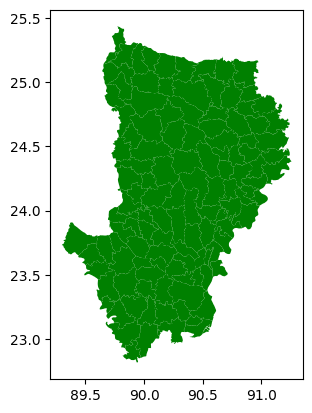So, can we make a map of Rajshahi district? We can, by considering the field 'NAME_2' field corresponding to district name.

In [ ]:
rajshahi = bd[bd['NAME_2'] == 'Rajshahi']
rajshahi.plot()

Out[ ]:
<Axes: >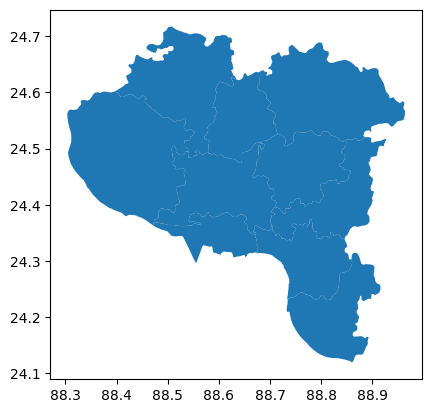Now, we will find out how many upazilas are in Sylhet division.

In [ ]:
sylhet_div = bd[bd['NAME_1'] == 'Sylhet'] # NAME_1 corresponds to division name

In [ ]:
sylhet_div.head()

Out[ ]:
ID_0 ISO NAME_0 ID_1 NAME_1 ID_2 NAME_2 ID_3 NAME_3 TYPE_3 ENGTYPE_3 NL_NAME_3 VARNAME_3 geometry
428 20 BGD Bangladesh 7 Sylhet 62 Hobiganj 429 Ajmiriganj Upazila|Thana|Po Sub-district NaN NaN POLYGON ((91.23438 24.56620, 91.23683 24.57435...
429 20 BGD Bangladesh 7 Sylhet 62 Hobiganj 430 Bahubal Upazila|Thana|Po Sub-district NaN NaN POLYGON ((91.48452 24.27334, 91.48559 24.27835...
430 20 BGD Bangladesh 7 Sylhet 62 Hobiganj 431 Baniachong Upazila|Thana|Po Sub-district NaN NaN POLYGON ((91.21724 24.42625, 91.22263 24.42789...
431 20 BGD Bangladesh 7 Sylhet 62 Hobiganj 432 Chunarughat Upazila|Thana|Po Sub-district NaN NaN POLYGON ((91.41032 24.11111, 91.40565 24.11828...
432 20 BGD Bangladesh 7 Sylhet 62 Hobiganj 433 Habiganj S. Upazila|Thana|Po Sub-district NaN NaN POLYGON ((91.33085 24.27030, 91.33200 24.27221...
In [ ]:
# Names of the upazilas
sylhet_div['NAME_3'].unique() # NAME_3 corresponds to upazila name

Out[ ]:
array(['Ajmiriganj', 'Bahubal', 'Baniachong', 'Chunarughat',
'Habiganj S.', 'Lakhai', 'Madhabpur', 'Nabiganj', 'Barlekha',
'Kamalganj', 'Kulaura', 'Maulvibazar S.', 'Rajnagar', 'Sreemangal',
'Bishwamvarpur', 'Chhatak', 'Derai', 'Dharampasha', 'Dowarabazar',
'Jagannathpur', 'Jamalganj', 'Sullah', 'Sunamganj S.', 'Tahirpur',
'Balaganj', 'Beanibazar', 'Bishwanath', 'Companiganj',
'Fenchuganj', 'Golabganj', 'Gowainghat', 'Jaintiapur', 'Kanaighat',
'Sylhet S.', 'Zakiganj'], dtype=object)
In [ ]:
# Number of upazila
len(sylhet_div['NAME_3'].unique())

Out[ ]:
35
In [ ]:
# Let's plot Bishwanath upazila
bishwanath = sylhet_div[sylhet_div['NAME_3'] == 'Bishwanath'] # subset the data to have data for Bishwanath upazila only
bishwanath.plot()

Out[ ]:
<Axes: >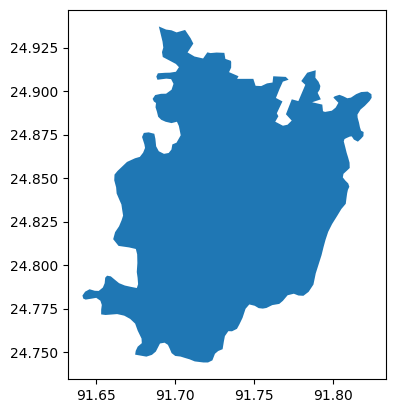In [ ]:
# Let's plot Chhatak upazila under Sylhet division
sylhet_div[sylhet_div['NAME_3'] == 'Chhatak'].plot()

Out[ ]:
<Axes: >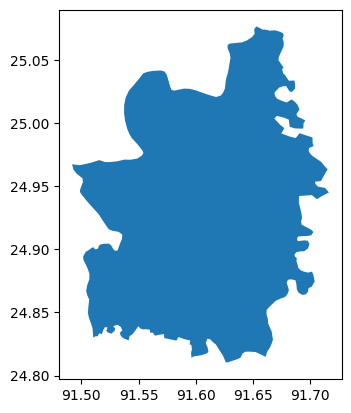We can use contextily package for adding basemaps.

In [ ]:
!pip install contextily

Looking in indexes: https://pypi.org/simple, https://us-python.pkg.dev/colab-wheels/public/simple/
Requirement already satisfied: contextily in /usr/local/lib/python3.10/dist-packages (1.3.0)
Requirement already satisfied: rasterio in /usr/local/lib/python3.10/dist-packages (from contextily) (1.3.6)
Requirement already satisfied: mercantile in /usr/local/lib/python3.10/dist-packages (from contextily) (1.2.1)
Requirement already satisfied: xyzservices in /usr/local/lib/python3.10/dist-packages (from contextily) (2023.2.0)
Requirement already satisfied: requests in /usr/local/lib/python3.10/dist-packages (from contextily) (2.30.0)
Requirement already satisfied: joblib in /usr/local/lib/python3.10/dist-packages (from contextily) (1.2.0)
Requirement already satisfied: pillow in /usr/local/lib/python3.10/dist-packages (from contextily) (8.4.0)
Requirement already satisfied: matplotlib in /usr/local/lib/python3.10/dist-packages (from contextily) (3.7.1)
Requirement already satisfied: geopy in /usr/local/lib/python3.10/dist-packages (from contextily) (2.3.0)
Requirement already satisfied: geographiclib<3,>=1.52 in /usr/local/lib/python3.10/dist-packages (from geopy->contextily) (2.0)
Requirement already satisfied: pyparsing>=2.3.1 in /usr/local/lib/python3.10/dist-packages (from matplotlib->contextily) (3.0.9)
Requirement already satisfied: packaging>=20.0 in /usr/local/lib/python3.10/dist-packages (from matplotlib->contextily) (23.1)
Requirement already satisfied: fonttools>=4.22.0 in /usr/local/lib/python3.10/dist-packages (from matplotlib->contextily) (4.39.3)
Requirement already satisfied: kiwisolver>=1.0.1 in /usr/local/lib/python3.10/dist-packages (from matplotlib->contextily) (1.4.4)
Requirement already satisfied: cycler>=0.10 in /usr/local/lib/python3.10/dist-packages (from matplotlib->contextily) (0.11.0)
Requirement already satisfied: contourpy>=1.0.1 in /usr/local/lib/python3.10/dist-packages (from matplotlib->contextily) (1.0.7)
Requirement already satisfied: python-dateutil>=2.7 in /usr/local/lib/python3.10/dist-packages (from matplotlib->contextily) (2.8.2)
Requirement already satisfied: numpy>=1.20 in /usr/local/lib/python3.10/dist-packages (from matplotlib->contextily) (1.22.4)
Requirement already satisfied: click>=3.0 in /usr/local/lib/python3.10/dist-packages (from mercantile->contextily) (8.1.3)
Requirement already satisfied: attrs in /usr/local/lib/python3.10/dist-packages (from rasterio->contextily) (23.1.0)
Requirement already satisfied: setuptools in /usr/local/lib/python3.10/dist-packages (from rasterio->contextily) (67.7.2)
Requirement already satisfied: cligj>=0.5 in /usr/local/lib/python3.10/dist-packages (from rasterio->contextily) (0.7.2)
Requirement already satisfied: click-plugins in /usr/local/lib/python3.10/dist-packages (from rasterio->contextily) (1.1.1)
Requirement already satisfied: affine in /usr/local/lib/python3.10/dist-packages (from rasterio->contextily) (2.4.0)
Requirement already satisfied: snuggs>=1.4.1 in /usr/local/lib/python3.10/dist-packages (from rasterio->contextily) (1.4.7)
Requirement already satisfied: certifi in /usr/local/lib/python3.10/dist-packages (from rasterio->contextily) (2022.12.7)
Requirement already satisfied: idna<4,>=2.5 in /usr/local/lib/python3.10/dist-packages (from requests->contextily) (3.4)
Requirement already satisfied: charset-normalizer<4,>=2 in /usr/local/lib/python3.10/dist-packages (from requests->contextily) (2.0.12)
Requirement already satisfied: urllib3<3,>=1.21.1 in /usr/local/lib/python3.10/dist-packages (from requests->contextily) (1.26.15)
Requirement already satisfied: six>=1.5 in /usr/local/lib/python3.10/dist-packages (from python-dateutil>=2.7->matplotlib->contextily) (1.16.0)

In [ ]:
import geopandas as gpd
import matplotlib.pyplot as plt
import contextily as ctx

# Set the figure size
fig, ax = plt.subplots(figsize=(10, 10))

# Plot the shapefile
bishwanath.plot(ax=ax, alpha=0.5)

# Add a basemap using contextily

/usr/local/lib/python3.10/dist-packages/contextily/tile.py:581: UserWarning: The inferred zoom level of 29 is not valid for the current tile provider (valid zooms: 0 - 19).